### Home > PC3 > Chapter 8 > Lesson 8.2.1 > Problem8-61

8-61.
1.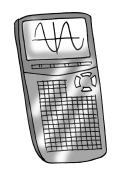There are no buttons for secant, cosecant, or cotangent on a calculator. In order to evaluate these expressions, the definition of the reciprocal function needs to be utilized. Use a calculator to evaluate each of the expressions below. The first answer is given as a check. All angles are in radians. Homework Help ✎

1. csc(3) ≈ 7.086

2. cot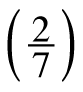3. sec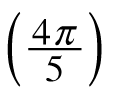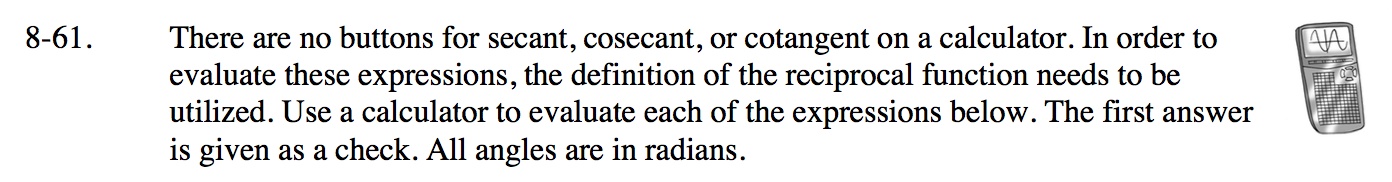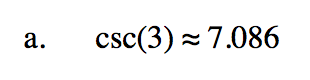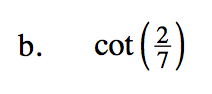$\frac{1}{\tan\left(\frac{2}{7}\right)}$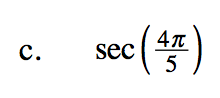$\frac{1}{\cos\left(\frac{4\pi}{5}\right)}$